# Younes

Hi! My name is Younes. I'm an electrical engineer and a renewable energy enthusiast. I created renewablewise.com with a mission of delivering digestible content and information to the people who seek it.

## What can a 200 watt solar panel power? A complete guide with examples.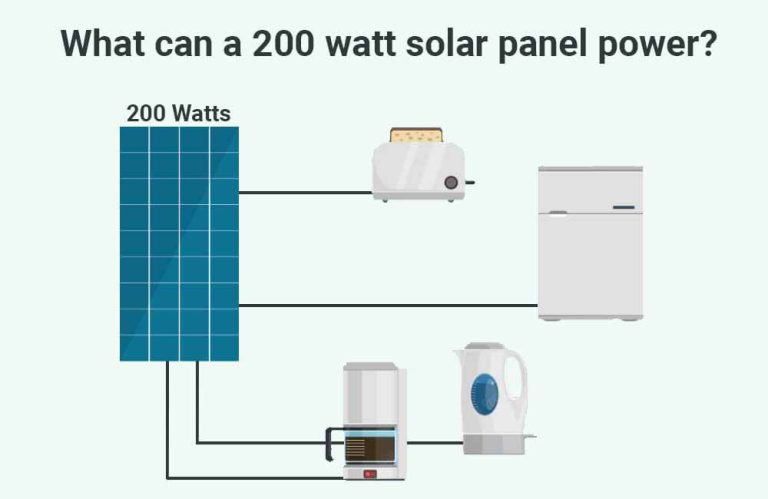In this article, I’ll help you put the electricity production capabilities of a 200 Watt solar panel into perspective, first, by answering some of the most frequently asked questions on the topic, such as: How much Electrical Current (Amps) does…

## How much power does a 300 Watt solar panel produce?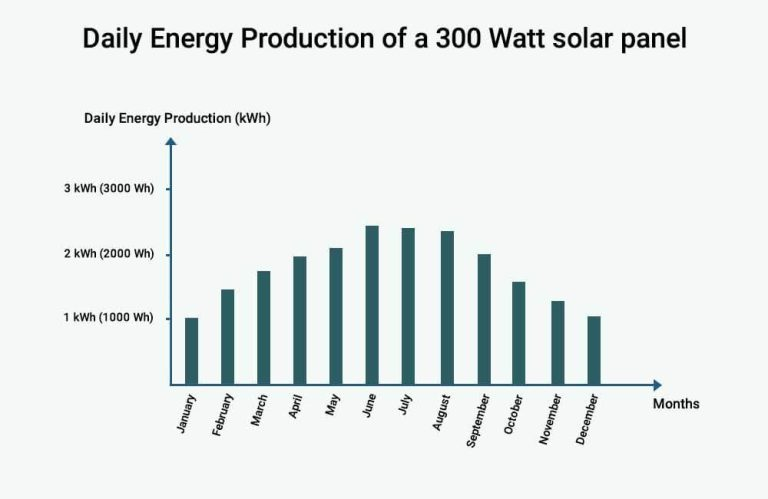Solar panels can be a pretty consistent source of energy, but when it comes to exactly how much energy that is, it is important to set your expectations right. To do that, it is important to understand the difference between…

## kW vs kWh: What is the difference between Power and Energy?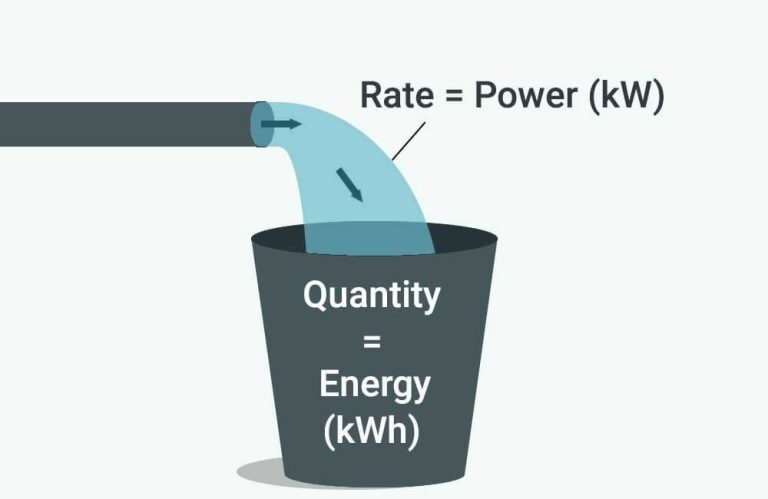Electricity measurement units can be very confusing and are often misunderstood, this is mainly because electricity itself is a complicated subject. In my article about Electricity and its measurement units, I discuss Amps, Volts, Watts, and Watt-hours. In this article,…

## Amps, Volts, Watts, and Watt-hours: Explaining electricity and its measurement unitsWhen you look at your utility bill, your electricity usage is measured in kWh, which stands for kiloWatt-hours (1000 Watt-hours) and is what your utility company charges you for at the end of the month. However, when you look at…

## Energy consumption calculator: Easily calculate the electricity usage of your appliances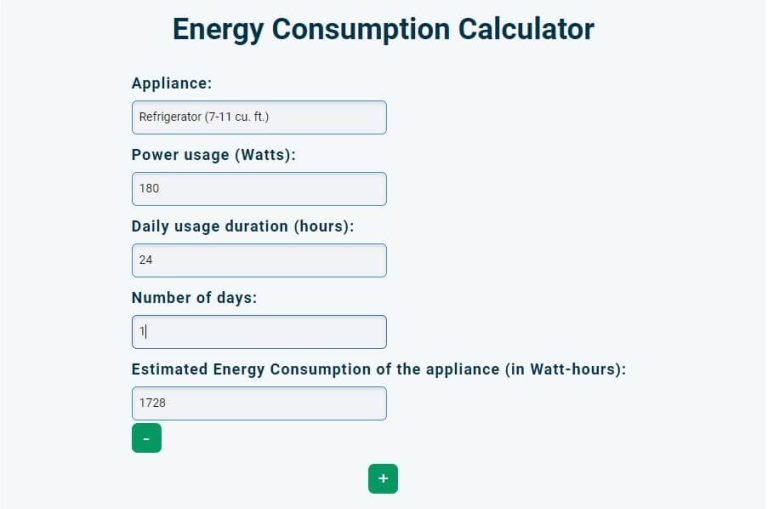Below, you’ll find our Energy Consumption Calculator, which helps you estimate your energy consumption in both Watt-hours (Wh) and kiloWatt-hours (kWh) based on: The appliances you use. Their Power Usage (in Watts). Their daily usage duration (in hours). The number…

## How much does a 5kw solar system produce?The 5kW (5000 Watts) rating on a solar system means that, provided enough direct sunlight, the system could potentially produce 5000 Watts of power. But the actual amount of power that a system of this size produces is not constant…

## How much electricity does a 4kw solar system produce?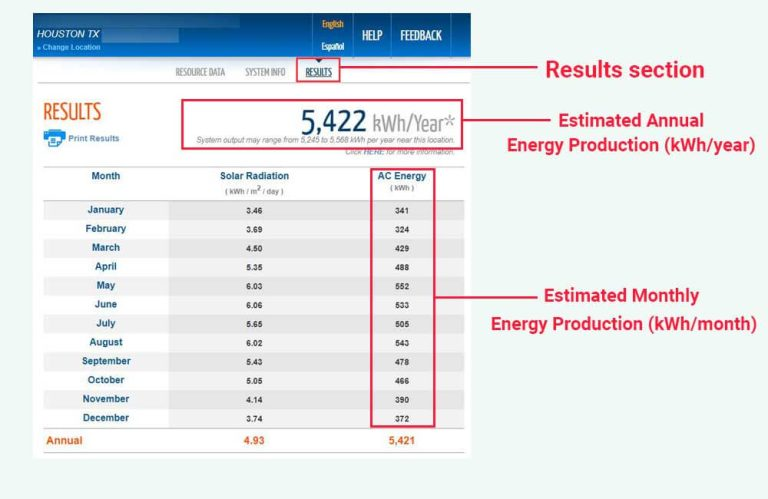Electricity has many measurement units, such as: Volts, which measure Electrical Potential, or simply voltage. Amps, which measure Electrical Current. Watts or kiloWatts, which measure Electrical Power. Watt-hours or kiloWatt-hours, which measure Electrical Energy. The 4kW (4000W) rating of a…

## How much power does a 3kw solar system produce?Unlike Energy, which is accumulative and is measured in Watt-hours (Wh) or kiloWatt-hours (kWh), Power is instantaneous and is measured in Watts (W) or kiloWatts (kW). Power measures the rate at which Energy is being generated. For example, a 3kW (3000…